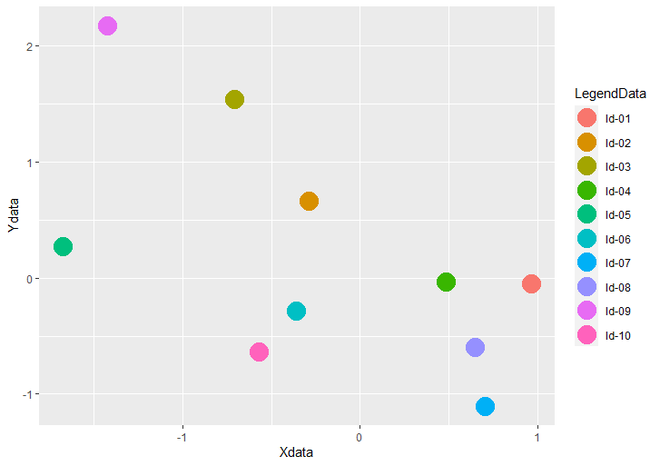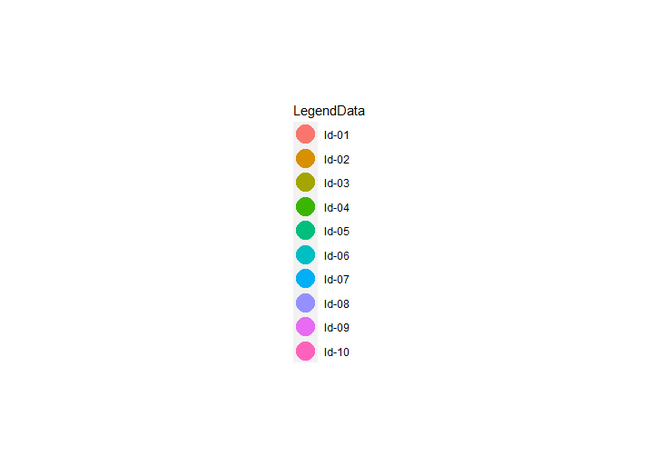Related Articles

# Draw ggplot2 Legend without Plot in R

• Last Updated : 23 May, 2021

A legend in the graph describes each part of the plot individually and is used to show statistical data in graphical form. In this article, we will see how to draw only the legend without a plot in ggplot2.

First, let us see how to draw a graph with a legend so that the difference is apparent. For that load the ggplot2 package by using library() function and create a DataFrame. To create an R plot, we use ggplot() function and for getting a scatter plot we add geom_point() function to ggplot() function.

Example:

## R

 `# Load Package``library``(``"ggplot2"``)`` ` `# Create a DataFrame ``data <- ``data.frame``(``  ``Xdata = ``rnorm``(10),                        ``  ``Ydata = ``rnorm``(10),``  ``LegendData = ``c``(``"ld-01"``, ``"ld-02"``, ``"ld-03"``,``                 ``"ld-04"``, ``"ld-05"``, ``"ld-06"``,``                 ``"ld-07"``, ``"ld-08"``, ``"ld-09"``, ``                 ``"ld-10"``))`` ` `# Create a Scatter Plot and assign it ``# to gplot data object``gplot <- ``ggplot``(data, ``aes``(``  ``Xdata, Ydata, color = LegendData)) +   ``  ``geom_point``(size = 7)``gplot`

Output:Scatter Plot with legend

### Packages Used:

Now, to draw only the legend of the plot without the plot, we have to load three packages named grid, gridExtra, and cowplot.

• grid package is used to produce a graphical output directly

Syntax:

install.packages(“grid”) #For Install grid package

• gridExtra is an extension to grid package, it supports the creation and editing of graphic objects but with higher-level functions than grid.

Syntax:

install.packages(“gridExtra”) #For Install gridExtra package

• cowplot package is used to enhance the presentability of figure by fixing its core components.

Syntax:

install.packages(“cowplot”) #For Install cowplot package

### Functions Used:

To draw only legend, we use three functions, which are from the above packages.

• get_legend() function is used to hold the legend to a plot.

Syntax : get_legend(ggplot)

Parameter: A ggplot, from which to retrieve the legend

Return : Legend from plot

• grid.newpage() moves the grid to another device by removing it from the former device.

Syntax : grid.newpage()

Return : Erases current plot window and create a new plot window

• grid.draw() produces graphical output from a graphical object

Syntax : grid.draw(legend)

Return : Draw the legend to new plot window

Thus, in order to draw a plot with just legend, first, a legend is drawn and held to the plot using get_legend(), then the plot is erased using grid.newpage() and then the legend is drawn to a new plot window using grid.draw().

Example:

## R

 `# Load Packages``library``(``"ggplot2"``)``library``(``"grid"``)``library``(``"gridExtra"``)``library``(``"cowplot"``)`` ` `# Create a DataFrame``data <- ``data.frame``(``  ``Xdata = ``rnorm``(10), Ydata = ``rnorm``(10),``  ``LegendData = ``c``(``"ld-01"``, ``"ld-02"``, ``"ld-03"``,``                 ``"ld-04"``, ``"ld-05"``, ``"ld-06"``,``                 ``"ld-07"``, ``"ld-08"``, ``"ld-09"``, ``                 ``"ld-10"``))`` ` `# Create a Scatter Plot``gplot <- ``ggplot``(data, ``aes``(Xdata, Ydata, color = LegendData)) +   ``  ``geom_point``(size = 7)`` ` `# Draw Only Legend without plot``# Grab legend from gplot``legend <- ``get_legend``(gplot)                    `` ` `# Create new plot window``grid.newpage``()                              `` ` `# Draw Only legend ``grid.draw``(legend) `

Output:Only Legend Without Plot

My Personal Notes arrow_drop_up